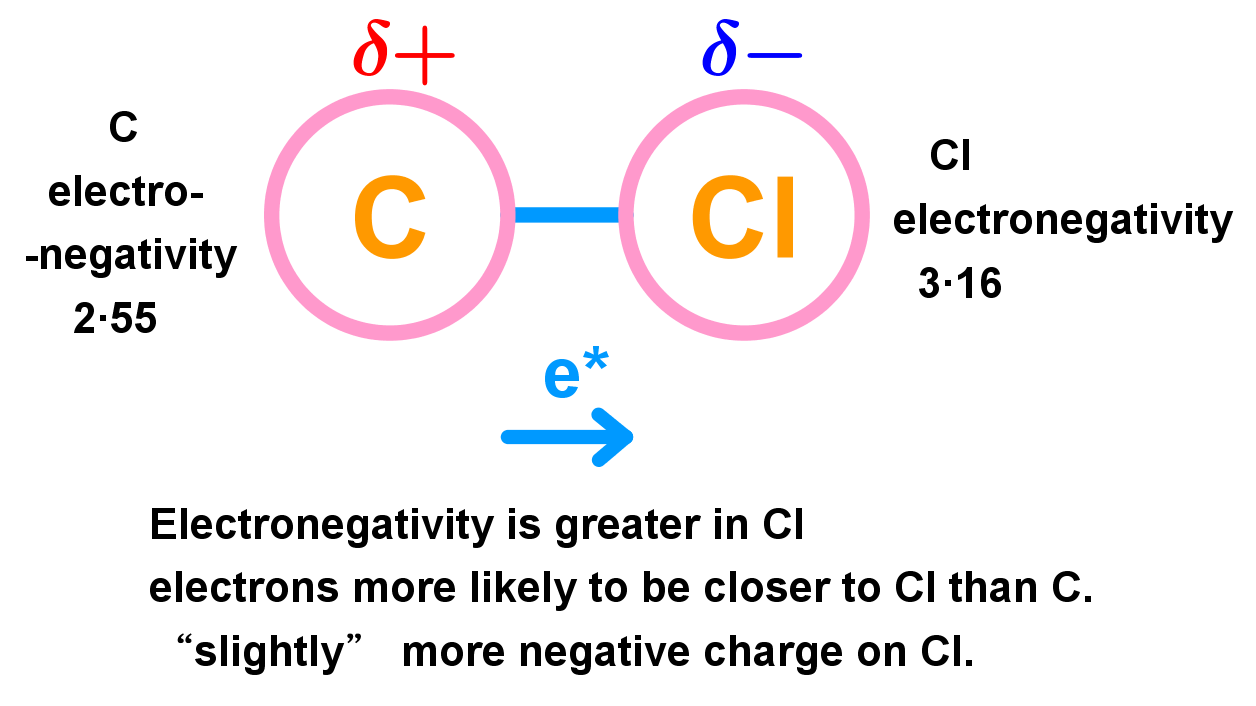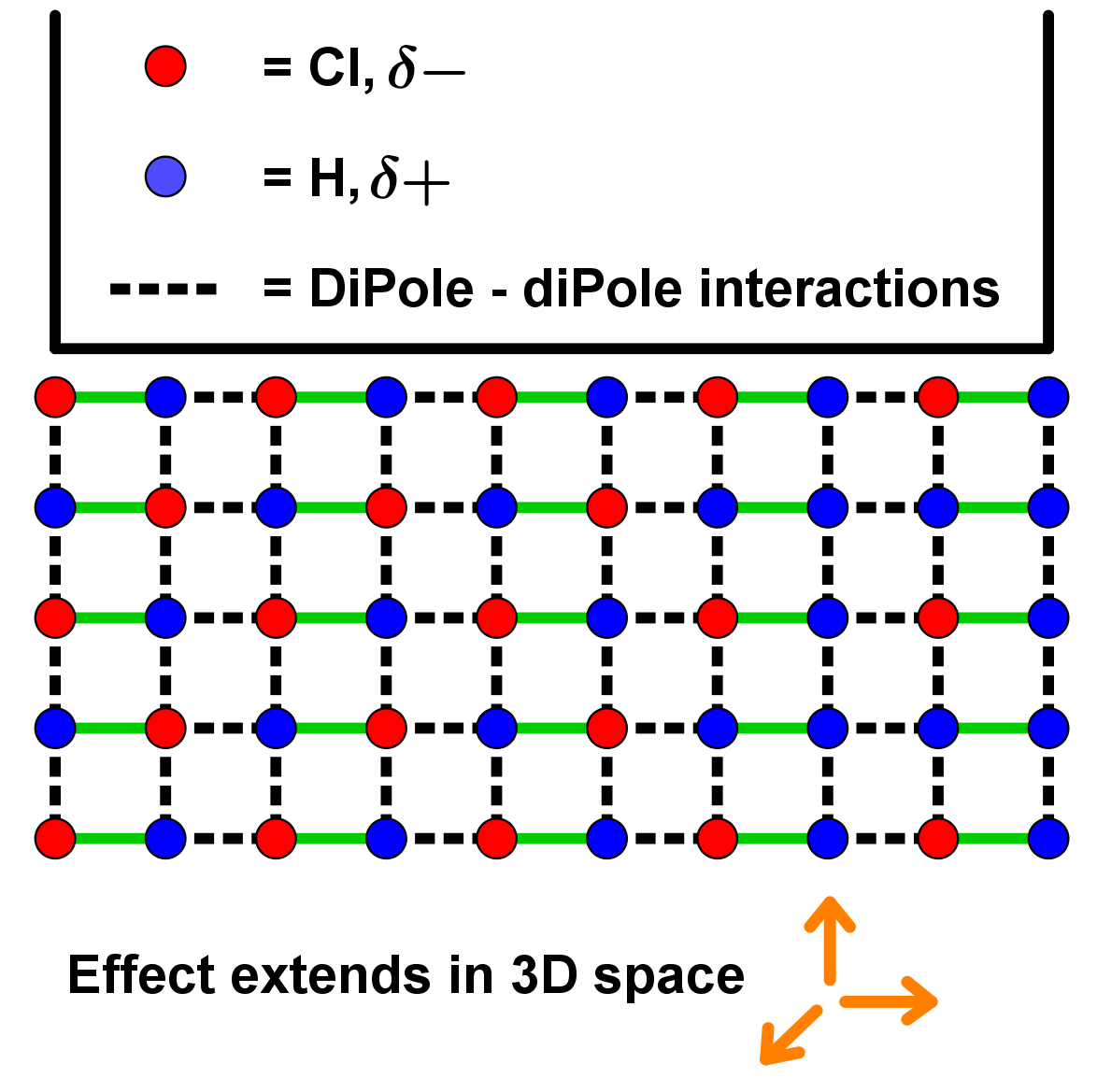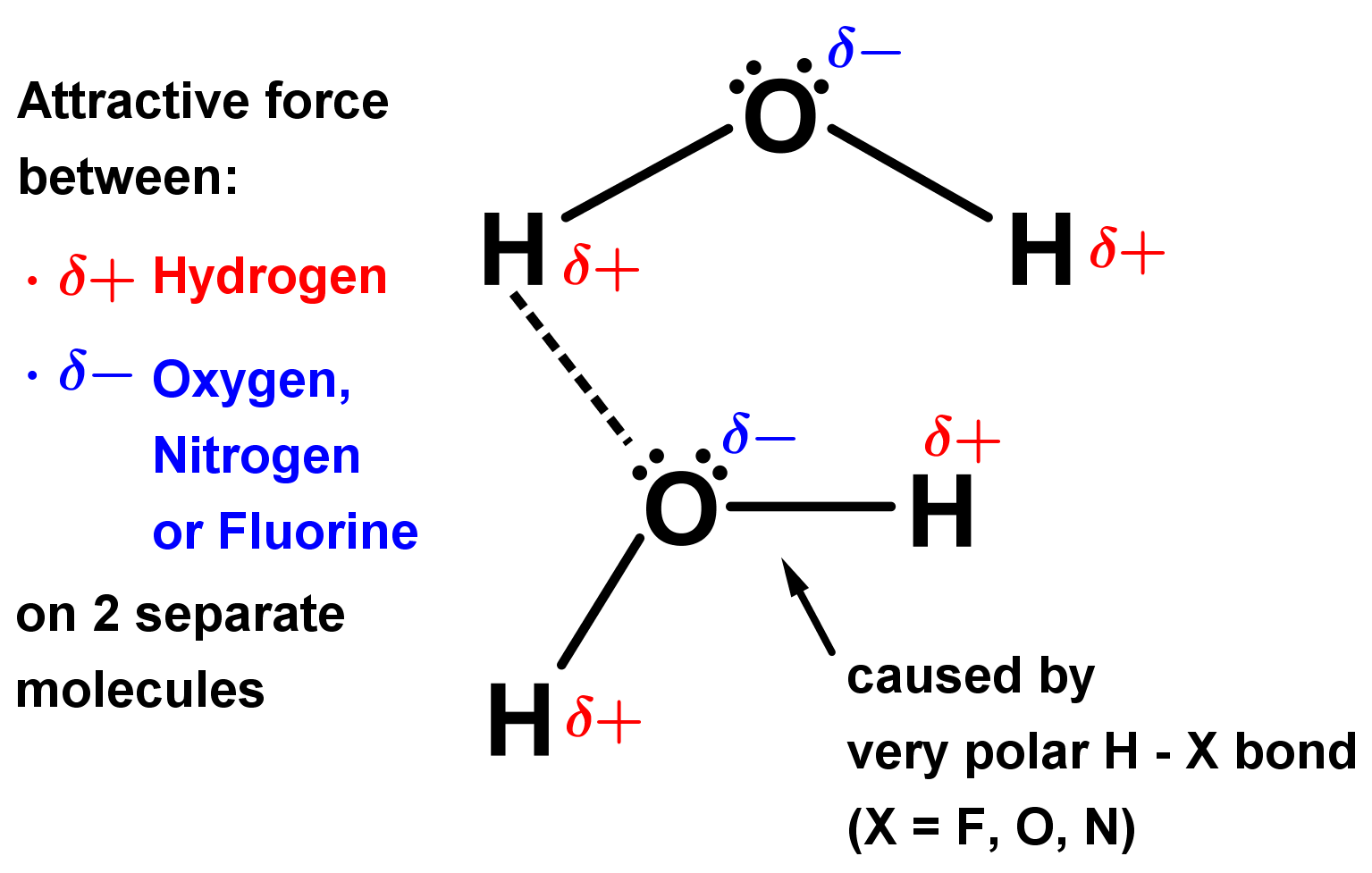# Intermolecular forces

### Intermolecular forces

#### Lessons

In this lesson, we will learn:
• To apply the principles of electrostatic forces to understand intermolecular forces.
• To explain how hydrogen bonding, London forces and dipole-dipole interactions occur.
• To recall the relative strength of relative forces between molecules.

Notes:
• The molecules of chemical compounds are held together by chemical bonds, or forces between the atoms the molecules are made of. As we saw earlier, these forces between atoms are called intramolecular forces and the two major types, like two ends of a left-to-right spectrum, are covalent and ionic bonding.

• As well as intramolecular forces, there are intermolecular forces that occur between the molecules of any chemical substances. Intermolecular forces are not as strong as intramolecular forces, but they influence a lot of properties in a chemical. For example, the melting point of a substance is greatly influenced by the intermolecular forces holding molecules together. To summarize, we have:
• Intramolecular forces, which are strong and hold the atoms within a molecule together. These are generally only broken in chemical reactions.
• The focus of this lesson: intermolecular forces, which are weaker but hold separate molecules together. These can generally be overcome by physical changes such as temperature.

• We now need to understand what a dipole is, because all intermolecular forces are based on dipole interactions. A dipole (di-pole, ‘two poles’) is a charge difference between atoms in part of a molecule, created by excess charge. Whenever an atom or molecule is more positive than another atom or molecule (which is more negative), you might have a dipole. This can happen in a few ways.
• Dipoles can form from a covalent bond between two elements of different electronegativity.
• Example below: carbon and chlorine can make a covalent bond. Because chlorine is more electronegative than carbon, the electron pair they share in the bond is going to be held closer to chlorine (on average) than carbon. This means that this part of the molecule on average is going to be slightly more negative (because the negatively-charged electrons are closer) around the chlorine atom than the carbon atom, where the relative lack of electrons leaves carbon with a slight positive charge. Therefore we give carbon a $\delta$+ (delta positive) and chlorine a $\delta$- (delta negative) charge. These are ‘partial’ positive / negative charges.
•• Because the electronegativity of an atom does not change, this effect will always be present in a carbon-chlorine bond – dipoles like this are called permanent dipoles. Any bond between two atoms with a difference in electronegativity creates a dipole like this. We call the bond a polar bond because it has the positive/negative charged effect of a north/south pole.

• A major type of intermolecular force are van der Waals forces:
• One type of van der Waals forces are dipole-dipole interactions.
• When a molecule of a compound has a permanent dipole (see above), the delta positive ( $\delta$+) and delta negative ( $\delta$-) charge influences other molecules around them too. This includes other identical molecules!
• Because each molecule has the same permanent dipole, they arrange with the opposite ends of other molecules to maximize their $\delta$+/ $\delta$- attractive forces with as many molecules as possible across 3d space. See below for an example with HCl, where H holds a $\delta$+ and Cl holds a $\delta$- charge in their covalent bond together.
•• Because this effect is caused by dipoles across molecules interacting, it is called dipole-dipole interactions. This is the stronger of the two van der Waals type forces.

• Another type of van der Waals forces are London dispersion forces (London forces).
• Because electrons repel each other and are attracted to positive charge, if two atoms or molecules approach one another, the electrons in each will repel the other. (See below: the solid black is high electron density, spotted is less electrons / lower density).
•• The movement of electrons due to repulsion polarizes both atoms and leads to a dipole being created: see above.
•• This 'forced' polarization of two atoms/molecules that come too close to each other is called an induced dipole. This is the attraction in London dispersion forces.
• Because this attractive force only exists when atoms/molecules are close together, and vanishes if they are moved apart, it is a temporary dipole, not a permanent one. For this reason we say London forces are temporary induced dipole forces. They are weaker than permanent dipole-dipole interactions (see above) and can exist between any atoms.
• London forces are stronger on atoms that are more polarizable – meaning the charge (electrons) on the atom can be manipulated and moved around. This occurs much more easily in larger atoms with more electron shells (so the outer shell is further from the nucleus). So, smaller atoms with less electron shells are less polarizable and have weaker London forces. Think of the difference between trying to twist and change the shape of a hard, small marble and a large bean bag – the bean bag is a more ‘polarizable’ larger atom!
• This explains why the melting point of helium is lowest in the noble gases, and melting point increases going down the group as London forces become stronger, needing more energy to overcome.

• Another type of intermolecular force is known as hydrogen bonding. These are the strongest intermolecular forces.
• Hydrogen bonding is a very strong form of dipole-dipole interactions that happens when hydrogen, H, covalently bonds to a very electronegative element ‘X’ (X = F, O or N). Because hydrogen is not very electronegative, these bonds make strong permanent dipoles; the $\delta$+ charge on hydrogen is relatively big, and the $\delta$- on the electronegative atom ‘X’ is equally strong.
• This causes attractive forces; interactions between the negative lone pair electrons on X in one molecule and the slightly positive hydrogen atom in the other. Chemists say the lone pair donates a hydrogen bond and the hydrogen atom accepts it. Hydrogen bonds are very similar to dipole-dipole interactions but even stronger.
• The properties affected by hydrogen bonding are cumulative; the more hydrogen bonding there is, the more the properties will are affected. Electronegative atoms with more than one lone pair (like oxygen) can donate more than one hydrogen bond, which affects properties more than if it could donate just one hydrogen bond (e.g. nitrogen).
•• Intermolecular forces are cumulative – they have an 'adding up' effect and will occur across all or any fraction of a molecule that makes contact with another. This is why:
• Longer hydrocarbon chains (with ‘more molecule’ to make contact) have a higher melting/boiling point than shorter ones.
• Unbranched straight hydrocarbon chains (which can get closer to one another, so they share more van der Waals forces) have a higher melting/boiling point than branched chains which can’t make as much contact.

• Water is a good example of how the strength and amount of hydrogen bonding in a chemical structure can change properties considerably:
• Compared to similar compounds with the same number of electrons, water has an unusually high melting/boiling point.
• With two electronegative lone pairs and two electropositive hydrogen atoms, it can donate two and accept two hydrogen bonds. With four hydrogen bonds per molecule in total, intermolecular forces are much stronger than in ammonia (only one lone pair and N-H being a weaker dipole) and methane (with no lone pairs and therefore no hydrogen bonding).
• Liquid water has a higher density than solid ice. That’s why icebergs float at the water’s surface!
• As a solid where hydrogen bonding dictates how the water molecules are ‘packed together’, the molecules are actually less compact than when they are liquid. This makes ice less dense and so it floats on the water’s surface

• A chemical substance’s solubility depends on the intermolecular forces it displays. This is important in planning chemical reactions. Generally, “like dissolves like” in terms of intermolecular forces between reactants and solvents. Some important points about solubility and intermolecular forces are below:
• The dipoles in water molecules are strong enough to dissolve some ionic compounds by interacting with the individual ions. The $\delta$- oxygen atoms can interact with positive ions, and $\delta$+ hydrogen atoms with negative ions. As solvent (water) molecules far outnumber ions, Multiple water molecules can surround ions in a process called hydration. This is why many simple ionic compounds are soluble in water.
• Alcohols such as ethanol are capable of hydrogen bonding, because of the O-H group present. Ethanol molecules will interact strongly with water molecules through their hydrogen bonding, so small simple alcohols such as ethanol are highly soluble in water through mutual hydrogen bonding.
• Not all polar molecules are soluble in water. Most haloalkanes for example have permanent dipoles due to the carbon-halogen bond. However, any dipole-dipole interactions they may have with water will not be strong enough to overcome interactions between hydrogen-bonding water molecules interacting among themselves. As such, haloalkanes are only slightly soluble in water because they cannot form the hydrogen bonds necessary to interact with a water solvent.

• The stronger or more intermolecular forces there are, the more the physical properties are affected – hardness, melting and boiling point and whether a substance conducts electricity are all affected by intermolecular forces. Later, in Polarity, you'll be able to predict these properties of substances based on intermolecular forces!
• Introduction
Intermolecular forces
a)
Definition of 'intermolecular force'

b)
Where intermolecular forces come from.

c)
Van der Waals: permanent dipole-dipole interactions.

d)
Van der Waals: London dispersion forces.

e)
Hydrogen bonding.

f)
Water and hydrogen bonding.

g)
Solubility and intermolecular forces.

h)
What influence do intermolecular forces have?

• 1.
Identify the intermolecular forces present in molecules.
Look at the formula of the chemical substances listed below. Which type of intermolecular forces would you expect to see between the molecules?
a)
i) CH$_4$
ii) H$_2$O

b)
i) Br$_2$
ii) HBr

• 2.
Recall the order of intermolecular force strength
Look at each group of chemical substances below. Order them in how relatively strong their intermolecular forces are. Hint: Identify the intermolecular forces present first.
a)
F$_2$, I$_2$, Br$_2$, Cl$_2$

b)
HCl, HF, HBr, HI

c)
NH$_3$, H$_2$O, CH$_4$